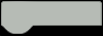# Arduino Temperature Control

This project is about control of temperature in values of 23 and 25 grades and control for hysteresis using DHT22 and Arduino 101.Things used in this project

### Hardware components

HARDWARE LIST
1 Gravity: 1602 LCD Keypad Shield For Arduino
1 Arduino 101
1 DHT22 Temperature Sensor
1 Jumper wires (generic)
2 LED (generic)
1 Resistor 10k ohm
2 Resistor 330 ohm

Arduino IDE

Story

### The Project

We want to show the operation of a temperature control system using hysteresis.### Components

The components we used were the following:### Temperature Control

The project does a temperature control, we model the following situation:

The system starts and shows the temperature, if the temperature reaches 25 degrees, then the green led is activated and the room starts to cool until the temperature drops to 23 degrees, the air conditioner turns off (red led) until the temperature rises again.And finally we have a video where you can see the operation:

Schematics

### Temperature controlCode

### Temperature control

Arduino

CODE
``````/*By Alexis Santiago Allende */
/*Last update: 12/03/2017*/
#include "DHT.h"//DHT sensor Library
#include <LiquidCrystal.h>//LCD Library
#define DHTPIN 12 //Define sensor pin(pin 12)
#define DHTTYPE DHT22 //What sensor use

DHT dht(DHTPIN, DHTTYPE);//Create sensor object
LiquidCrystal lcd(8, 9, 4, 5, 6, 7);//Create lcd object using this pins 8,9,4,6,7

boolean a=LOW,b=HIGH;
float h=0,t=0;
const int ledPin = 3; //LED pins for temperature control
const int ledPin2 = 2;
long previousMillis = 0;
long interval = 2000; //Read sensor each 2 seconds

void setup() {
lcd.begin(16, 2);              //Start lcd
lcd.setCursor(0,0);            //Set in position 0,0
// set the digital pin as output:
pinMode(ledPin, OUTPUT);//Change to output my pins
pinMode(ledPin2, OUTPUT);
dht.begin();//Start DHT22 sensor
digitalWrite(ledPin2,LOW);//Turn off LED
digitalWrite(ledPin,LOW);//Turn off LED
lcd.print("Tempera:");// print in lcd this word
lcd.setCursor(0,1);//Change cursor position
lcd.print("Humedad:");// print in lcd this word
}

void loop()
{
unsigned long currentMillis = millis();//time elapsed
if(currentMillis - previousMillis > interval) //Comparison between the elapsed time and the time in which the action is to be executed
{
previousMillis = currentMillis; //"Last time is now"

//Below is for print data sensors in lcd
lcd.setCursor(10,0);
lcd.print(t);
lcd.setCursor(10,1);
lcd.print(h);

if(t>=25 && a==LOW)//if temperature above of 25 degrees
{
digitalWrite(ledPin,HIGH);//Active air conditioner
digitalWrite(ledPin2,LOW);
a=HIGH;
b=LOW;

}
else if(t<=23&&b==LOW)//if temperature is under 23 degrees
{
digitalWrite(ledPin2,HIGH);
digitalWrite(ledPin,LOW);//Turn off air conditioner
a=LOW;
b=HIGH;
}

}
}``````

The article was first published in hackster, March 12, 2017